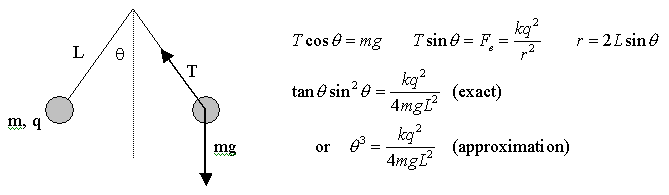### Simple Electroscope : Equal-mass like-charged pendulum bobs hanging from common point

This physlet illustrates a dynamic method to find the answer to a complicated equation. In an electroscope problem (see explanation below physlet), if you have the angle, and need to find the charge required, it is quite easy to solve. But, if you have the charge, and need to figure out the angle - there is no closed form solution (but there is an approximate solution).

 This physlet shows an intial electroscope setup - you select the charges, the length of the string, and the masses. The system will then calculate an angle based on the approximation outlined below. It will set the angle for the electroscope, and then "let it go". But, depending on the quality of the approximation, the charges might not be in equilibrium, so they will start to move (basically oscillate around the equilibrum).        Then, you can use the "damp velocity" button to temporarily halt the system at any chosen point in time (pretend you grab onto the charges to stop them, and then let them go again). If you damp the velocity at the right times, you will eventually find the correct equilibrium angle. This angle could be put back into the original complete equation, to test to see if the correct magnitude of charge results! Set the starting values :        Length (cm) =            Mass (kg) =            Charge (microCoulombs) =            ---------------------------------------------------   * If you manually change values ...

#### Introduction to the theory :

This is a model of a simple electroscope - the two "bobs" will separate from each other because they have like charges. The separation will depend on the length of the strings, the mass of the bobs, and how much charge is on the bobs. We can use simple forces to generate the equations we would need to model this system :Now, to solve the exact equation, we would need to use something like MAPLE - there is no easy analytical solution. Here is a Maple worksheet that calculates this model, and let's you change values. (Right click on the link, and use the "save as" choice to copy the worksheet to your computer, then open it in Maple.)

In the animation above, there are two like-charged 500-gram spheres attached to strings to form an electroscope (position given in centimeters and time is in seconds).  You may drag the spheres to whatever position you wish before or during the animation. Now, initially, the system will use the approximate angle to place the masses, based on the choices you make to the right of the animation. For small angles, the approximate initial angle is very good, so the system will essentially be static (that is, it is the correct angle for equilibrium). But, if the angle is big (such as if the length is small, and the charge is big) - the approximate angle will be different enough from the exact angle, that the system will "oscillate" a little. If this happens, you can damp the motion by clicking the "Damp Velocity" button. (It will temporarily stop the charges from moving .. as they get close to their equilibrium points, periodically "damp" them, until they are motionless .. thus, in equilibrium).
Reset using current values
Reset using original values when you loaded the page

Credits: Physlet problem authored by Mario Belloni. Modified by Scott Schneider at LTU.